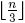# On irrationality of some distances between points on a circle

Notes on Number Theory and Discrete Mathematics
Print ISSN 1310–5132, Online ISSN 2367–8275
Volume 22, 2016, Number 2, Pages 4—9

## Details

### Authors and affiliations

Mladen Vassilev–Missana5 Victor Hugo Str, Ap. 3
1124 Sofia, Bulgaria

### Abstract

Let n > 3 be arbitrary integer. In the present paper it is shown that if K is an arbitrary circle and Mi, i = 1,…,n are points on K, dividing K into n equal arcs, then for each point M on K, different from the mentioned above, at leastof the distances |MMi| are irrational numbers.

### Keywords

• Distance
• Irrational number
• Circle

• 97G40
• 11XX

### References

1. Coolidge, J. L. (1939) A Historically Interesting Formula for the Area of a Quadrilateral. Amer. Math. Monthly, 46, 345–347.
2. Nagell, T. (1951) Introduction to Number Theory. Wiley, New York.
3. Mason, J. C., & Handscomb, D. C. (2003) Chebyshev Polynomials. CRC Press, New York.

## Cite this paper

Vassilev–Missana, M. (2016). On irrationality of some distances between points on a circle. Notes on Number Theory and Discrete Mathematics, 22(2), 4-9.